# Creating basic calculated metrics and attributes

Follow

In the Calculation engine menu (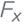), you can create a variety of calculated metrics and attributes. To get started, you can create standard calculated metrics and attributes. For a list of all available calculated metrics and attributes, see Calculation engine reference.

## Standard calculated metrics

With calculated metrics, you can create calculations based on any field in the dataset with a wide range of functions. For example, you can use basic mathematical operators to calculate profit from your base revenue and cost metrics.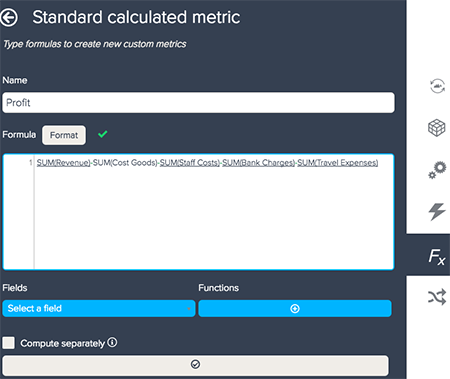When you begin to enter a formula, BIME will suggest a match to the metric and aggregator. For a list of functions you can add to your formulas see, Formula writing rules.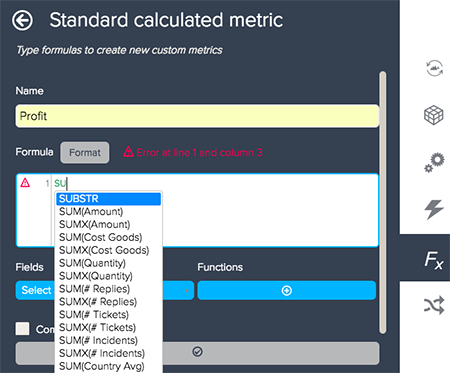You can also add attributes to your formulas by selecting them from the Select a field drop-down list. Any calculated attributes such as global variables will appear.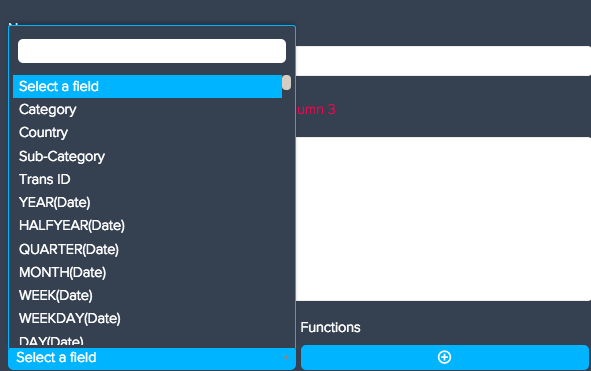Lastly, you can add functions to your formula. Functions will provide specific actions for your data. For a list of functions you can add to your formulas, see Formula writing rules.

1. In one of the calculation options, click the +Add button underneath Functions.
2. Select a function from the list of available functions. You can also use the Filter button to show functions for only selected categories.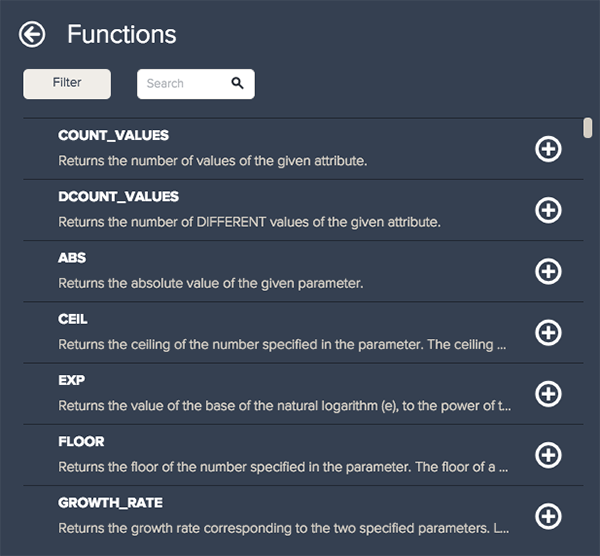3. Click the + button next to a function to add it to the formula.

## Standard calculated attributes

Just like calculated metrics, calculated attributes provide maximum flexibility. You can use your dataset's attributes and metrics to create new attributes, whether they are groups, categories, labels, filters, etc. A calculated attribute contains all the same functionalities as a calculated metric, but with a couple additions.

The Computed from drop-down list contains all available attributes to use as a foundation. This does not include global variables. These attributes can still be added as a field as well.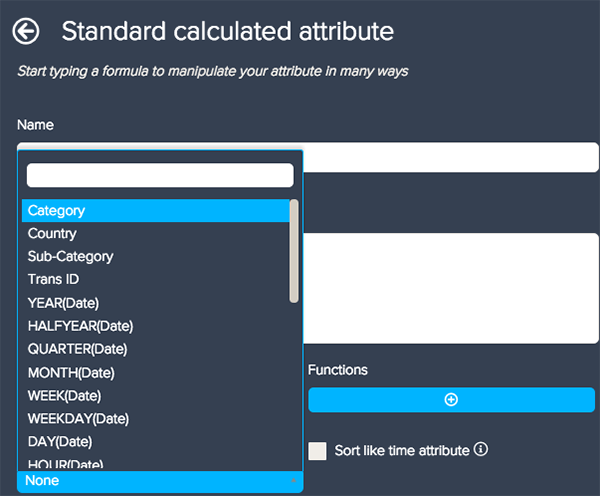You can also check the Sort like time attribute box, to sort your date values chronologically. If BIME recognizes date elements, it will automatically place them in the correct order.

0 out of 2 found this helpful
Have more questions? Submit a request

• Jessica, is there a way you know of to generate a filter to only show an attribute where its measure is positive? Like, only show campaigns where cost > 0?

•Jessica Marasco

Hey Eric,

You can use the IF THEN ELSE function for this (see Writing IF THEN ELSE logical functions). You can try creating a standard calculated attribute using the formula below:

IF SUM(Cost)>0 THEN [Campaigns] ENDIF

If your cost or campaigns measure and attributes are called something different, replace with the correct names. You can then add the standard calculated attribute and your normal Cost measure to your query.

• Hi,

I'm not able to calculate the following formula for some reason:

SUM(money)/DCOUNT_VALUES([client])

i suspect it's because i'm using Dcount.. but why?

you urgent response is much appreciated!